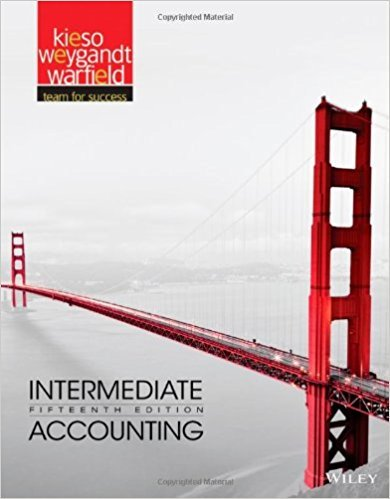×
×

# Solutions for Chapter 4: Intermediate Accounting 15th Edition## Full solutions for Intermediate Accounting | 15th Edition

ISBN: 9781118147290Solutions for Chapter 4

Solutions for Chapter 4
4 5 0 399 Reviews
21
3
##### ISBN: 9781118147290

Intermediate Accounting was written by and is associated to the ISBN: 9781118147290. This expansive textbook survival guide covers the following chapters and their solutions. Since 37 problems in chapter 4 have been answered, more than 7043 students have viewed full step-by-step solutions from this chapter. This textbook survival guide was created for the textbook: Intermediate Accounting, edition: 15. Chapter 4 includes 37 full step-by-step solutions.

Key Business Terms and definitions covered in this textbook
• average tax rate

total taxes paid divided by total income

• budget surplus

an excess of tax revenue over government spending

the failure of majority rule to produce transitive preferences for society

• externality

the uncompensated impact of one person’s actions on the wellbeing of a bystander

• income effect

the change in consumption that results when a price change moves the consumer to a higher or lower indifference curve

• inflation

an increase in the overall level of prices in the economy

• internalizing the externality

altering incentives so that people take account of the external effects of their actions

• law of demand

the claim that, other things being equal, the quantity demanded of a good falls when the price of the good rises

• lump-sum tax

a tax that is the same amount for every person

• marginal product of labor

the increase in the amount of output from an additional unit of labor

• median voter theorem

a mathematical result showing that if voters are choosing a point along a line and each voter wants the point closest to his most preferred point, then majority rule will pick the most preferred point of the median voter

• microeconomics

the study of how households and firms make decisions and how they interact in markets

• model of aggregate demand and aggregate supply

the model that most economists use to explain shortrun fluctuations in economic activity around its long-run trend

• Nash equilibrium

a situation in which economic actors interacting with one another each choose their best strategy given the strategies that all the other actors have chosen

• quantity demanded

the amount of a good that buyers are willing and able to purchase

• quantity theory of money

a theory asserting that the quantity of money available determines the price level and that the growth rate in the quantity of money available determines the inflation rate

• random walk

the path of a variable whose changes are impossible to predict

• reserve ratio

the fraction of deposits that banks hold as reserves

• unit of account

the yardstick people use to post prices and record debts

• variable costs

costs that vary with the quantity of output produced

×